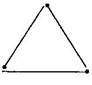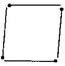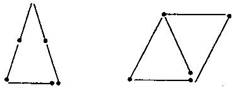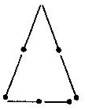### Understanding Elementary Shapes-Solutions Ex-5.6

CBSE Class –VI Mathematics
NCERT Solutions
Chapter 5 Understanding Elementary Shapes (Ex. 5.6)

Question 1. Name the types of following triangles:
(a)Triangle with lengths of sides 7 cm, 8 cm and 9 cm.
(b)$\mathrm{\Delta }\text{ABC}$ with AB = 8.7 cm, AC = 7 cm and BC = 6 cm.
(c)$\mathrm{\Delta }$PQR such that PQ = QR = PR = 5 cm.
(d)$\mathrm{\Delta }$DEF with $m\mathrm{\angle }$D = ${90}^{\circ }$
(e)$\mathrm{\Delta }$XYZ with $m\mathrm{\angle }$Y = ${90}^{\circ }$ and XY = YZ
(f)$\mathrm{\Delta }$LMN with $m\mathrm{\angle }$L = ${30}^{\circ },$$m\mathrm{\angle }$M = ${70}^{\circ }$ and $m\mathrm{\angle }$N = ${80}^{\circ }.$
(b) Scalene triangle
(c) Equilateral triangle
(d) Right-angled triangle
(e) Isosceles right-angled triangle
(f) Acute-angled triangle
Question 2. Match the following:
 Measure of Triangle Types of Triangle (i)3 sides of equal length (a) Scalene (ii) 2 sides of equal length (b) Isosceles right angle (iii) All sides are of different length (c) Obtuse angle (iv) 3 acute angles (d) Right angle (v) 1 right angle (e) Equilateral (vi) 1 obtuse angle (f) Acute angle (vii) 1 right angle with two sidesof equal length (g) Isosceles

Answer: (i) $\to$ (e), (ii) $\to$ (g), (iii) $\to$ (a), (iv) $\to$ (f), (v) $\to$ (d), (vi) $\to$ (c), (vii) $\to$ (b)
Question 3. Name each of the following triangles in two different ways: (You may judge the nature of angle by observation)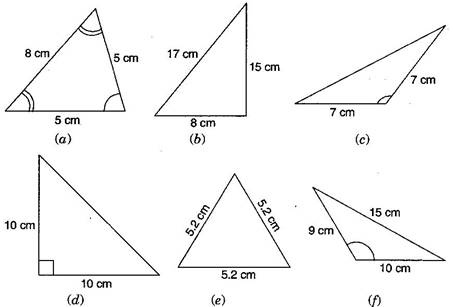Answer: (a) Acute angled triangle and Isosceles triangle
(b) Right-angled triangle and Scalene triangle
(c) Obtuse-angled triangle and Isosceles triangle
(d) Right-angled triangle and Isosceles triangle
(e) Equilateral triangle and acute angled triangle
(f) Obtuse-angled triangle and scalene triangle
Question 4. Try to construct triangles using match sticks. Some are shown here.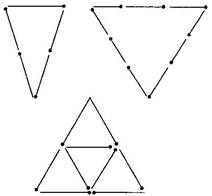Can you make a triangle with:
(a) 3 matchsticks?
(b) 4 matchsticks?
(c) 5 matchsticks?
(d) 6 matchsticks?
(Remember you have to use all the available matchsticks in each case)
If you cannot make a triangle, think of reasons for it.